Next: 5.2 Discretization with Tetrahedrons Up: 5. Discretization with the Previous: 5. Discretization with the

Subsections

# 5.1 Basics

The basic aim of the finite element method is to solve a PDE, or a system of coupled PDE s, numerically. Instead of finding the analytic solution of the PDE, which is usually a function of the coordinates, it is tried to determine this function values for discrete coordinates on grid points. For this purpose the continuum is discretized with a number of so-called finite elements which results in a mesh with grid nodes. If the finite elements are appropriately small, the solution of the PDE can be approximated with a simple function, the so-called shape function, in each element, which acts as a contribution to the approximation of the global solution of the PDE. All together a linear or non-linear equation system must be obtained. The real advantage is that such (non-)linear equation systems can be quite easily solved today by computer programs.

## 5.1.1 Mesh Aspects

For solving a PDE numerically, in the first step the simulation domain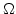is divided into a number of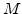(as possible geometrically simple) elements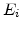(like tetrahedrons or cubes). The quality of the approximated solution depends on the size and so on the number of finite elements in the discretized domain. The elements must be located in such a manner, that there are no empty spaces between them and that they do not overlap: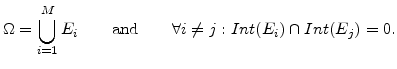(5.1)

Here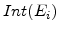is the set of all points in the element, except those which are located on the surface. Furthermore, surface conformity of neighbor elements is demanded. This means that on each surface of an element inside the domain exact only one neighbor element is bordered.

If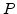is the set of all grid nodes of the discretized domain, than each grid node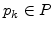has a unique global index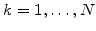.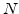is the number of all nodes in the whole mesh. A node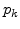can also have several local indices.

## 5.1.2 Shape Function

From the mathematical point of view the shape function shall interpolate the discrete solution function values between the grid nodes. If a PDE is written in the form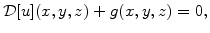(5.2)

where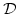is a second order differential operator, the desired solution of the PDE is a function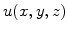, which is approximated by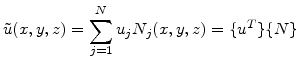(5.3)

The summation is performed over all grid nodes. Thereby,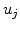is the respective value of the function at a grid node with the number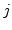and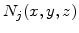is the shape function.

The shape function can be chosen quite freely, but it must be appropriate for interpolation. In addition every shape functionmust have a value of 1 on the grid node j (with coordinates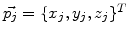) and values of 0 on all other grid nodes: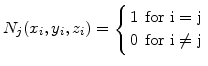(5.4)

In practical applications linear shape functions or polynomials of low order are used. The numbering of the grid nodes should be carried out in a way, that nodes at Dirichlet-boundaries, where the solution ofis already known, are ranked behind the others. The nodes where the valuemust be calculated get the indices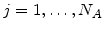, the other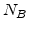nodes at the Dirichlet-boundaries are indexed with.

In order to get the solution of the PDE, only'' the unknown coefficientsin (5.3) must be obtained. For determining the valuesthere are two available ways, the method of Ritz or the method of weighted residuals . The method of Ritz seeks a stationary point of the variational functional . This variational approach leads to an integral formulation of the PDE. The Ritz method can only be applied, if for the boundary problem an equivalent variational formulation exists. The more universal method is based on the weighted residuals and therefore it is used in this work.

## 5.1.3 Weighted Residual Method

When the approximated solution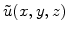is reinserted in the PDE (5.2), there exists a residual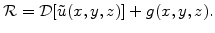(5.5)

If the residual would disappear (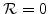), the exact solution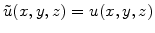would have been found . Since the function space of the approximation solutions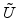is a subset of the function space of the exact solutions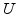, such a solution does not exist for the general case. Therefore, it is tried to fulfill the residual condition not exactly, but withweighted or averaged linearly independent weight functions (also called test functions)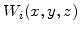, so that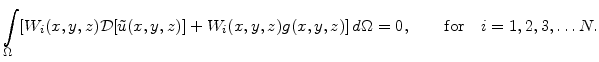(5.6)

The weight functions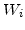must be chosen in a suitable way, because the quality of the solution depends on them. If the weight functions are identical with the shape functions (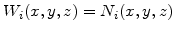), this approach is called Galerkin's method .

With the approximated solution (5.3) the weighted residual (5.7) can be rewritten in the form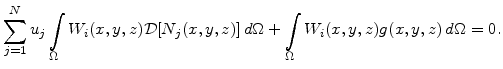(5.7)

It is possible to determine theunknown function valueson the grid nodes with this system ofequations.Next: 5.2 Discretization with Tetrahedrons Up: 5. Discretization with the Previous: 5. Discretization with the

Ch. Hollauer: Modeling of Thermal Oxidation and Stress Effects